# History

Euler's formula was proven for the first time by Roger Cotes in 1714 in the form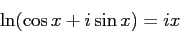(where "ln" means natural logarithm, i.e. log with base e).[John Stillwell (2002). Mathematics and Its History. Springer.]

Euler published the equation in its current form in 1748, basing his proof on the infinite series of both sides being equal. Euler considered it natural to introduce students to complex numbers much earlier than we do today. In his elementary algebra text book, Elements of Algebra, he introduces these numbers almost at once and then uses them in a natural way throughout.

The appeal to me from the first time I saw it in the form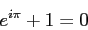is that it provides a link between two fundamental constants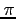and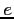, the numbers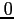and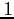and the wholly imaginarg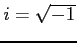. This is often referred to as Euler's identity. There are many more uses of this amazing relationship and the book Dr. Euler's Fabulous Formula: Cures Many Mathematical Ills. by Paul J. Nahin (Princeton University Press (10 April 2006) ISBN-10: 0691118221), is worth reading.

For a proof of Euler's Formula by Julius O. Smith III see

http://ccrma-www.stanford.edu//~jos//mdft//Proof_Euler_s_Identity.html


danny 2009-08-22